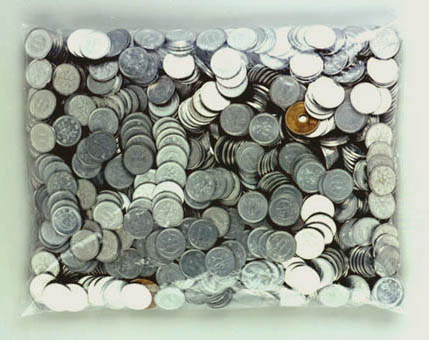to english | index | next

1184枚の硬貨から成る1210円 (金額第一三番)
1210 Yen Which Consists of 1184 Coins (Money Amount No. 13)

Japanese coins, a plastic bag
2001
photo by Takagi Shigeki

cf. 1184 and 1210 are the amicable pair which Paganini found in 1866.
(Two numbers are called amicable if each equals to the sum of the divisors of the other.)
Divisors of 1184: 1 + 2 + 4 + 8 + 16 + 32 + 37 + 74 + 148 + 296 + 592 = 1210
Divisors of 1210: 1 + 2 + 5 + 10 + 11 + 22 + 55 + 110 + 121 + 242 + 605 = 1184